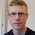## Friday, 18 August 2017

### The Final Polish

We are preparing for releasing version 3.0.0 of the interval package and this last week have mainly been about fixing minor bugs related to the release. I mention two of the more interesting bugs here.

### Compact Format

We (Oliver) recently added support for "format compact" when printing intervals. It turns out that the way to determine if compact format is enabled differs very much between different version of Octave. There are at least three different ways to get the information.

In the older releases (< 4.2.0 I believe) you use "get (0, "FormatSpacing")" but there appear to be a bug for version < 4.0.0 for which this always return "loose".

For the current tip of the development branch you can use "[~, spacing] = format ()" to get the spacing.

Finally in between these two version you use "__compactformat__ ()".

In the end Oliver, probably, found a way to handle this mess and compact format should now be fully supported for intervals. The function to do this is available here https://sourceforge.net/p/octave/interval/ci/default/tree/inst/@infsup/private/__loosespacing__.m.

### Dot-product of Empty Matrices

When updating "dot" to support N-dimensional arrays I also modified it so that it behaves similar to Octaves standard implementation. The difference is in how it handles empty input. Previously we had

> x = infsupdec (ones (0, 2));
> dot (x, x)
ans = 0×2 interval matrix

but with the new version we get

> dot (x, x)
ans = 1×2 interval vector
_com   _com

which is consistent with the standard implementation.

In the function we use "min" to compute the decoration for the result. Normally "min (x)" and "dot (x, x)" returns results of the same size (the dimension along which it is computed is set to 1), but they handle empty input differently. We have

> x = ones (0, 2);
> dot (x, x)
ans =
0   0
> min (x)
ans = [](0x2)

This meant that the decoration would be incorrect since the implementation assumed they always had the same size. Fortunately the solution was very simple. If the dimension along which we are computing the dot-product is zero. the decoration should always be "com". So just adding a check for that was enough.

You could argue that "min (ones (0, 2))" should return "[inf, inf]" similarly to how many of the other reductions, like "sum" or "prod", return their unit for empty input. But this would most likely be very confusing for a lot of people. And it is not compatible with how Matlab does it either.

## Updates on the Taylor Package

I have also had some time to work on the Taylor package this week. The basic utility functions are now completed and I have started to work on functions for actually computing with Taylor expansions. At the moment there are only a limited amount of functions implemented. For example we can calculate the Taylor expansion of order 4 for the functions $\frac{e^x + \log(x)}{1 + x}$ at $x = 5$.

## Create a variable of degree 4 and with value 5
> x = taylor (infsupdec (5), 4)
x = _com + _com X + _com X^2 + _com X^3 + _com X^4

## Calculate the function
> (exp (x) + log (x))./(1 + x)
ans = [25.003, 25.004]_com + [20.601, 20.602]_com X + [8.9308, 8.9309]_com X^2 + [2.6345, 2.6346]_com X^3 + [0.59148, 0.59149]_com X^4

1.Seeing the Taylor package in action with this nice example is great! I guess that this is the first implementation of Taylor arithmetic with decorated intervals, isn't it?

2.I don't know of any other implementation. INTLAB only supports Taylor arithmetic using undecorated intervals.

3.This is the first implementation as far as I'm aware to.

I think the decoration could play a critical role for some algorithms. For example Newtons interval method required that the function is continuous on the domain, with decorated intervals this could be checked